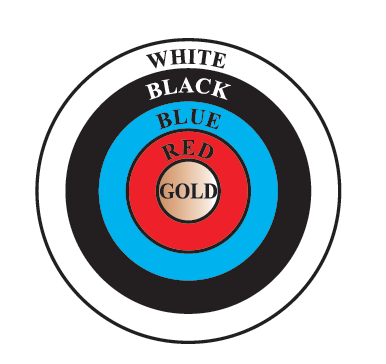# Ex.12.1 Q3 Areas Related to Circles Solution - NCERT Maths Class 10

Go back to  'Ex.12.1'

## Question

Given figure depicts an archery target marked with its five scoring areas from the centre outwards as Gold, Red, Blue, Black and White. The diameter of the region representing Gold score is $$21 \,\rm{cm}$$ and each of the other bands is $$10.5 \,\rm{cm}$$ wide. Find the area of each of the five scoring regions.

[Use $$\pi= \,\frac{22}{7}$$]Video Solution
Areas Related To Circles
Ex 12.1 | Question 3

## Text Solution

What is known?

Diameter of the gold region and width of the other regions.

What is unknown?

Area of each scoring region.

Reasoning:

Area of the region between $$2$$ concentric circles is given by \begin{align}\pi {\text{r}}_2^2 - \pi {\text{r}}_1^2\end{align}.

Steps:

Radius$$({r_1})$$ of gold region (i.e., $$1^\rm{st}$$ circle)$$= \frac{{21}}{2} = 10.5\,{\text{cm}}$$

Given that each circle is $$10.5\,\rm{cm}$$ wider than the previous circle.

Therefore,

Radius $$(r_2)$$ of $$2^\rm{nd}$$ circle

\begin{align}&= 10.5 + 10.5\\&=21 \,\rm{cm}\end{align}

Radius $$({r_3})$$ of $$3^\rm{rd}$$circle

\begin{align}&= 21 + 10.5\\ &= 31.5\,{\text{cm}}\end{align}

Radius $$(r_4)$$ of $$4^\rm{th}$$ circle

\begin{align}&= 31.5 + 10.5\\ &= 42\,{\text{cm}}\end{align}

Radius $$(r_5)$$ of $$5^\rm{th}$$ circle

\begin{align}&= 42 + 10.5\\ &= 52.5\,\,{\text{cm}} \end{align}

Area of gold region$$=$$  Area of $$1^\rm{st}$$ circle $$= \pi {r}_1^2 = \pi {(10.5)^2} = 346.5\;\rm{cm^2}$$

Area of red region $$=$$Area of $$2^\text{nd }$$circle $$-$$ Area of $$1^\rm{ st }$$circle

\begin{align}& = \pi {\text{r}}_2^2 - \pi {\text{r}}_1^2\\& = \pi {{(21)}^2} - {{(10.5)}^2}\\& = 441\pi - 110.25\pi = 330.75\pi \\& = 1039.5\,{\text{c}}{{\text{m}}^2}\end{align}

Area of blue region $$=$$ Area of $$3^\text{rd}$$ circle $$-$$ Area of $$2^\rm{nd}$$circle

\begin{align}&= \pi _{13}^2 - \pi {\text{r}}_1^2\\& = \pi {{(31.5)}^2} - \pi {{(21)}^2}\\&= 992.25\pi - 441\pi = 551.25\pi \\&= 1732.5\,{\text{c}}{{\text{m}}^2}\end{align}

Area of black region$$=$$ Area of $$4^\rm{th}$$ circle $$-$$Area of $$3^\rm{rd}$$circle

\begin{align}& = \pi r_4^2 - \pi r_3^2\\& = \pi {{(42)}^2} - \pi {{(31.5)}^2}\\&= 1764\pi - 992.25\pi \\&= 771.75\pi \\ &= 2425.5\,{\text{c}}{{\text{m}}^2}\end{align}

Area of white region $$=$$ Area of $$5^\rm{th}$$ circle $$-$$ Area of $$4^\rm{th}$$circle

\begin{align}&= \pi {\text{r}}_5^2 - \pi \pi _4^2\\&= \pi {{(52.5)}^2} - \pi {{(42)}^2}\\&= 2756.25\pi - 1764\pi \\&= 992.25\pi \\ &= 3118.5\,{\text{c}}{{\text{m}}^2}\end{align}

Therefore,areas of gold, red, blue, black, and white regions are $$346.5\, \rm{cm^2},$$$$1039.5 \,\rm{cm^2},$$ $$1732.5 \,\rm{cm^2},$$ $$2425.5\,\rm{cm^2},$$and$$3118.5 \,\rm{cm^2}$$ respectively.

Learn from the best math teachers and top your exams

• Live one on one classroom and doubt clearing
• Practice worksheets in and after class for conceptual clarity
• Personalized curriculum to keep up with school